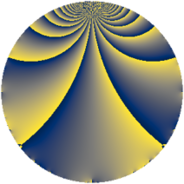# Properties

 Label 3724.1.bkLevel $3724$ Weight $1$ Character orbit 3724.bk Rep. character $\chi_{3724}(1451,\cdot)$ Character field $\Q(\zeta_{6})$ Dimension $4$ Newform subspaces $1$ Sturm bound $560$ Trace bound $0$

# Related objects

## Defining parameters

 Level: $$N$$ $$=$$ $$3724 = 2^{2} \cdot 7^{2} \cdot 19$$ Weight: $$k$$ $$=$$ $$1$$ Character orbit: $$[\chi]$$ $$=$$ 3724.bk (of order $$6$$ and degree $$2$$) Character conductor: $$\operatorname{cond}(\chi)$$ $$=$$ $$532$$ Character field: $$\Q(\zeta_{6})$$ Newform subspaces: $$1$$ Sturm bound: $$560$$ Trace bound: $$0$$

## Dimensions

The following table gives the dimensions of various subspaces of $$M_{1}(3724, [\chi])$$.

Total New Old
Modular forms 44 20 24
Cusp forms 12 4 8
Eisenstein series 32 16 16

The following table gives the dimensions of subspaces with specified projective image type.

$$D_n$$ $$A_4$$ $$S_4$$ $$A_5$$
Dimension 0 4 0 0

## Trace form

 $$4q - 4q^{4} - 2q^{6} + O(q^{10})$$ $$4q - 4q^{4} - 2q^{6} - 2q^{13} + 4q^{16} - 2q^{17} - 2q^{22} + 2q^{24} - 4q^{25} - 2q^{29} - 4q^{33} - 2q^{37} - 4q^{38} + 2q^{41} + 2q^{46} + 2q^{52} + 4q^{54} - 2q^{57} + 2q^{61} - 2q^{62} - 4q^{64} + 2q^{68} + 4q^{69} - 2q^{73} - 2q^{78} + 2q^{81} + 2q^{86} + 2q^{88} - 2q^{89} - 4q^{93} + 2q^{94} - 2q^{96} - 2q^{97} + O(q^{100})$$

## Decomposition of $$S_{1}^{\mathrm{new}}(3724, [\chi])$$ into newform subspaces

Label Dim. $$A$$ Field Image CM RM Traces $q$-expansion
$$a_2$$ $$a_3$$ $$a_5$$ $$a_7$$
3724.1.bk.a $$4$$ $$1.859$$ $$\Q(\zeta_{12})$$ $$A_{4}$$ None None $$0$$ $$0$$ $$0$$ $$0$$ $$q+\zeta_{12}^{3}q^{2}+\zeta_{12}q^{3}-q^{4}+\zeta_{12}^{4}q^{6}+\cdots$$

## Decomposition of $$S_{1}^{\mathrm{old}}(3724, [\chi])$$ into lower level spaces

$$S_{1}^{\mathrm{old}}(3724, [\chi]) \cong$$ $$S_{1}^{\mathrm{new}}(532, [\chi])$$$$^{\oplus 2}$$